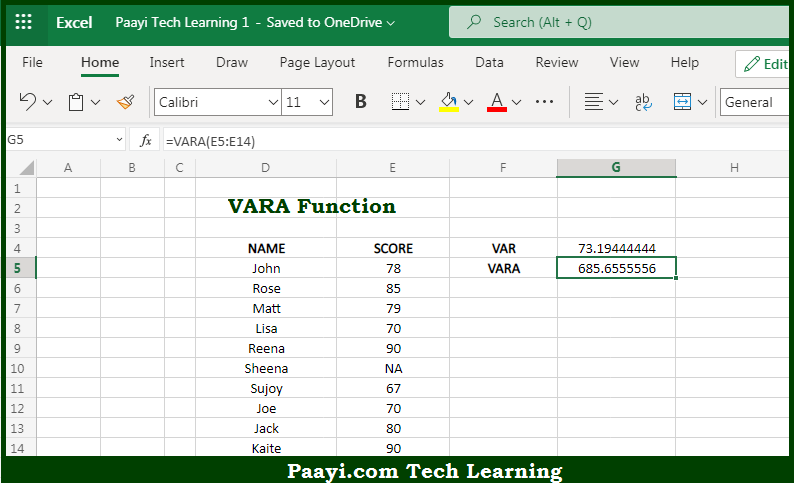# Learn How to Use Microsoft Excel VARA Function

Written by | 0 Comments | 607 Views

In this article, you will learn how to use the Microsoft Excel VARA function and its prime function in Microsoft Excel. You will also get to know the Microsoft Excel VARA function return value and syntax with the help of some examples.

Microsoft Excel VARA Function

The main purpose of the Microsoft Excel VARA function is to get the variation of the sample or population. That implies, with the help of the VARA function you can able to estimate the variance of the sample of a given data set or population. It should be noted that the VARA function evaluates text values and the logical in references, unlike the VAR function. So, with the help of the VARA function, you can able to get the variation of the sample or population.

Return Value of VARA Function

The return value will be the calculated variance.

Syntax of VARA Function

=VARA(number1, [number2], ...)

Where the arguments:

• number1: This is the first number or reference.
• number2: This is the second number or reference (optional).

## How to Use Microsoft Excel VARA Function?So we know that Microsoft Excel VARA function you can able to get the variation of the sample or population. That implies, with the help of the VARA function you can able to estimate the variance of the sample of a given data set or population. It should be noted that the VARA function evaluates text values and the logical in references, unlike the VAR function. So, with the help of the VARA function, you can able to get the variation of the sample or population.